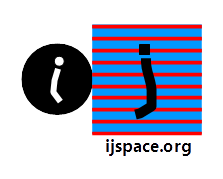Stitching Up the Measurement Space Together - III 2nd November 2019 HOME "The interpretation of quantum mechanics has been dealt with by many authors, and I do not want to discuss it here. I want to deal with more fundamental things." - Dirac "Until now, the only planets whose formation was compatible with disc instabilities were a handful of young, hot and very massive planets far away from their host stars. With GJ 3512b, we now have an extraordinary candidate for a planet that could have emerged from the instability of a disc around a star with very little mass. This find prompts us to review our models." - Hubert Klahr,  Max Planck Institute for Astronomy. https://www.bbc.com/news/science-environment-49855058 *** Let us consider a simple interaction, between an electron and a photon, resulting in a change in momentum of the electron due to absorption of the photon, and hence a change in the field as shown below: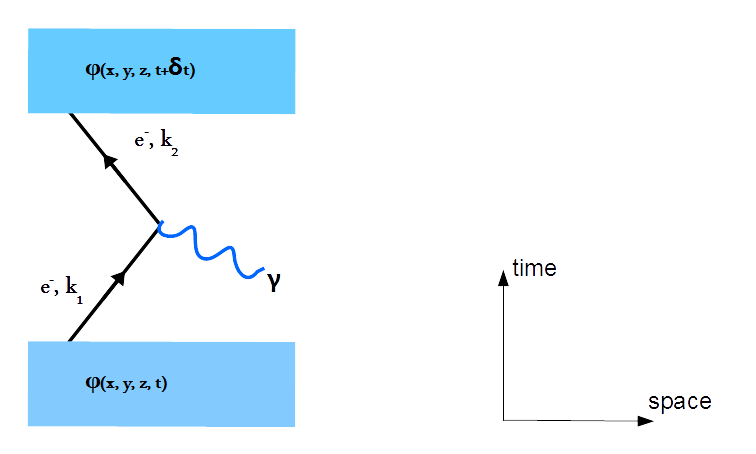The picture shown above represents the "coupling" between an electron and a photon.  Other possibility is the emission of a photon by an electron as its momentum is changed, as shown below: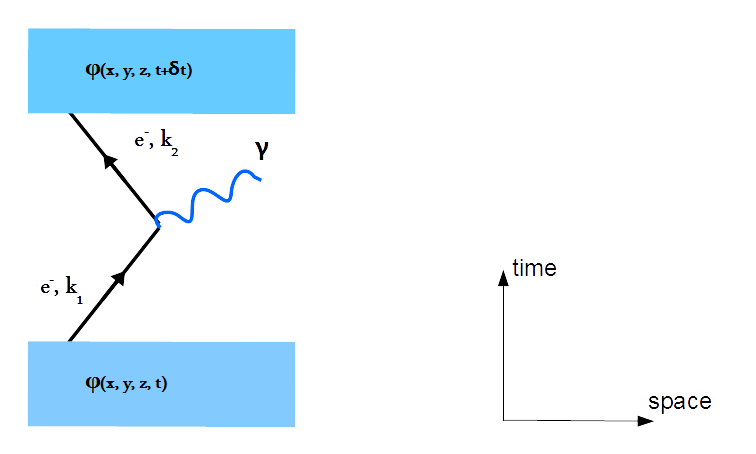However the actual process of an interaction is not as bare-bones as shown here, where a single electron (e) and a single photon (γ), are assumed to exist.  In Quantum Electrodynamics (QED), to accurately estimate the effect of a simplest e-γ interaction, we must include the effects of the vacuum polarization and the electron-positron pair formation.  Subsequently there are infinite possible paths for the simplest e-γ interaction.  We already know that in a discrete measurement space or j-space, a perfect point particle can not be measured.        We are showing below a representative diagram based on QED concepts, with the conventional time axis along the horizontal line, for the field-change with respect to time, for an arbitrary point in space in the discrete measurement space.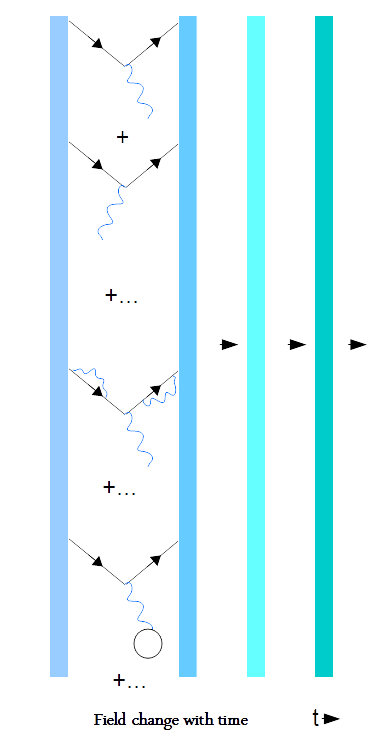Ideally each of these infinite paths must be measured by Aku's Measurement System (AMS), to properly characterize the simplest electron-photon interaction.  As it happens, AMS does not have this capability, only the precision observers, Obscs (v/c ~ 1, 133Cs, HeNe), do.   In other words, the measurement space and AMS for Aku (ObsM), is established by Obscs as discussed previously.     Please note that to simplify  the discussion, so far we are not accounting for the change in physical location (x, y, z) as the momentum of the electron changes, due to its interaction with the photon.  However the uncertainty principle requires change in position corresponding to the change in momentum of the interacting electron.  Thus the picture based on the basic electron-photon interaction in j-space looks as follows: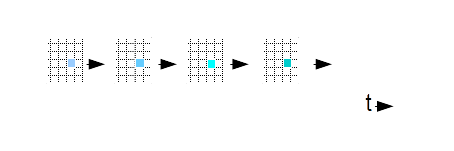In above picture each "j-pixel" represents the change in field  at a "point" with respect to time, in j-space.   (The shape of j-pixel is of no consequence to us.)  The progression of two neighbouring j-pixels  with  time is shown  below: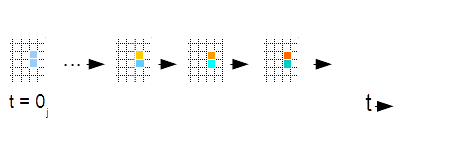So what do we mean by "stitching the j-space together"?  We have to seamlessly stitch two neighbouring j-pixels at an arbitrary instant on the time-axis of an epoch.  And if we think carefully, Lorentz Invariance allows us to do so.  Both neighbouring j-pixels are bound by Lorentz Invariance.  And since Lorentz Transformation and Möbius Transformation are equivalent, at a given instant, the structures measured in the neighbouring pixels can be transformed into each other using Möbius transformations.      We can easily extrapolate this statement to all the j-pixels possible in space and time for a given epoch, by stating that in Aku's measurement space, they are "seamlessly" connected to each other by Lorentz and Möbius transformations in time and space1.  Furthermore there are likely to be many-body interactions between various elementary particles, aka Hartree-Fock picture, which must be accounted for before we can describe field φ  at a point (x, y, z) at time instants t and t+δt, as measured by AMS for Aku (ObsM).  Please note that the progression of an individual j-pixel with respect to time is irreversible, as entropy in j-space is continuously increasing.     Before proceeding further, we must mention that the discussion above, corresponds to the exterior region-1 of Kruskal-Szekeres Coordinates.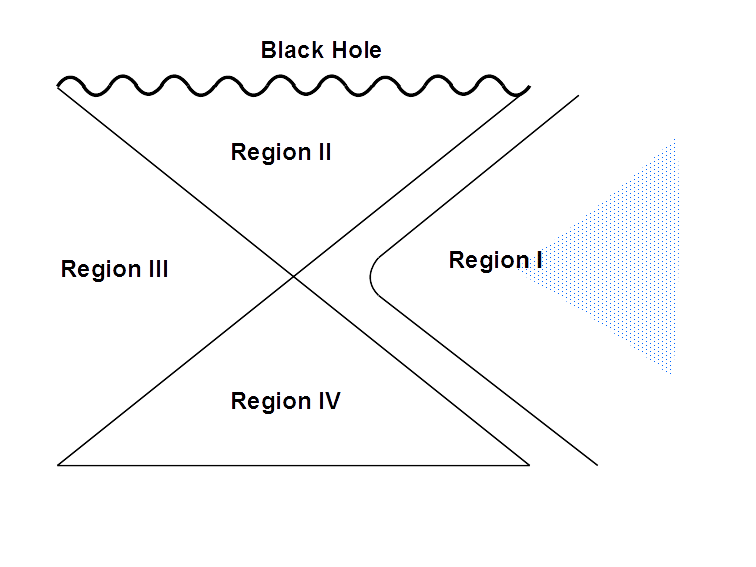We also note that since the picture we are developing is based on measurements by AMS using electron-photon interaction, we are essentially in Fermi space.     Discussion so far has been strictly limited to the structure of the measurement space based on a simple e-γ interaction.  We assume this j-space structure as the basic fabric of space-time, and try to describe the various physical structures embedded into it.  Again the question maybe asked that in the information space what do we mean by "various physical structures embedded into the discrete measurement space".  The situation is as follows: The information structures and subsequent physical structures are represented by δ-functions, where each delta function has its own definition for zeros and infinities.  In a simplified picture unified by Lorentz Invariance, we can assume zeros and infinities to be identical for various physical structures (Newtonian Mechanics).  However for more complex information structures, renormalization procedure has to be used to account for the redefinition of the integration limits.  The picture shown above translates into different scenarios in the physical world as follows2: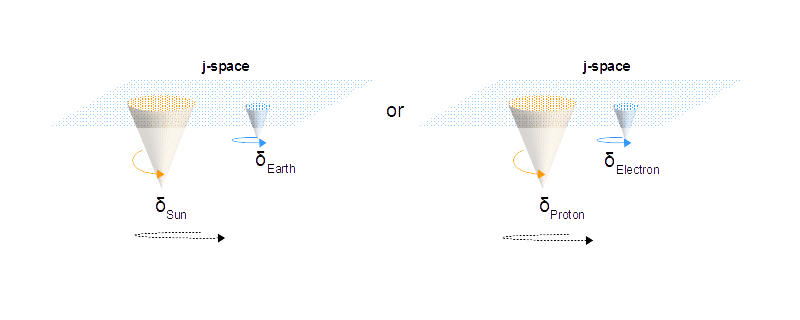The advantage of the description developed in a discrete measurement space, is quite evident.   The cosmic and elementary structures,  both  correspond to the measurements made in j-space.  Therefore the physical laws developed for discrete measurement space, will be universally true, independent of the physical picture, they are applied to.  This procedure is far more simpler than trying to unify the physical laws corresponding to different information spaces, i.e. Cosmic (Gravitation) and Elementary (Nuclear, Quantum etc.).  The nature of force in each of these pictures, would depend upon the interaction used to make measurements in j-space.     Another advantage, is the presence of the curvature in the information space due to δ-functions.  The quantity "mass", is a measured quantity in space time.  The nature of mass is different for each quadrant in Kruskal-Szekeres Coordinates. While some of the complex δ-functions are likely to have corresponding higher mass and hence the curvature in space-time (region-I), it is also possible that more complex δ-functions, can not be measured at all due to the relativistic nature of corresponding higher order information spaces.  Such structures may have very small measured mass, but they will have extraordinarily strong pull similar to gravitation.  (We are moving away from the idea that the inertial-mass measured in region-1, alone is responsible for the curvature in space-time.  Aku's physical universe correspond to the measurements, only in region-1.)     Finally, we will briefly discuss how to develop the mathematical structures based on the measurements performed in j-space.  We use Hamilton's principle for a given path in the measurement space as follows:In above equation, the interval element ds, is defined as,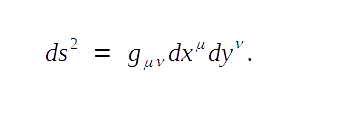The metric tensor gμν contains the information about the j-space and thus the nature of the interaction.  In j-space we refrain from assigning known coordinate frames to dx and dy variables3.  The geodesic is used to rewrite Hamilton's principle, in presence of the physical structures or equivalently information space δ-functions as: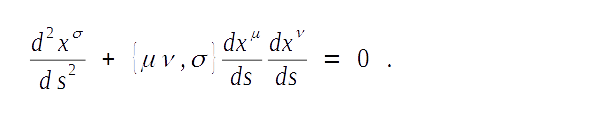The quantity {μν, σ} is known as Christoffel three-index symbols and it represents the effect of the information δ-functions on j-space fabric, as its curvature near information sources.  In the absence of the information structures, {μν, σ} = 0 and j-space is flat.  Furthermore per Obsc measurements ds = 0j.  We can visualize the Hamilton's principle for the familiar space-time description, as follows: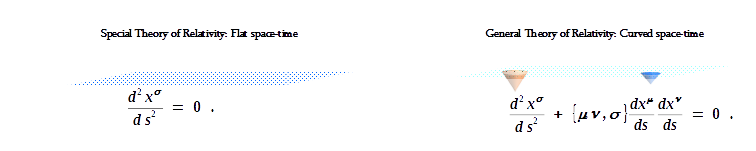We note that for light rays, ds = 0.  In j-space the equivalent condition is that for Obsc, ds = 0j, i.e. ds is zero for 133Cs, and HeNe, but per Obsi criterion ds is finite4.  Finally we also note that multiple measurement fabrics corresponding to different interaction mechanisms within j-space, will exist as shown below: In each of these j-space fabrics, Christoffel three-index symbols, {μν, σ}, would have different values.  We will  discuss the introduction of information structures, ethereal and corporal, into the j-fabric next.  ___________________ 1.  In j-space what is seamless for ObsM and Obsc, is granular for Obsi. 2. The j-space can not be either irrotational or source-free.  We have discussed this aspect of the discrete measurement space before.  3. We will discuss the requirements for the coordinate frames, based on j-space measurements later on.  The description based on conventional coordinate systems such as spherical or cylindrical, has limited effectiveness. 4. Obsi criterion in j-space is equivalent to Maxwell's demon in thermodynamics.  We should also think how does the entropy of the measurements in j-space, affect the value of 0j? ***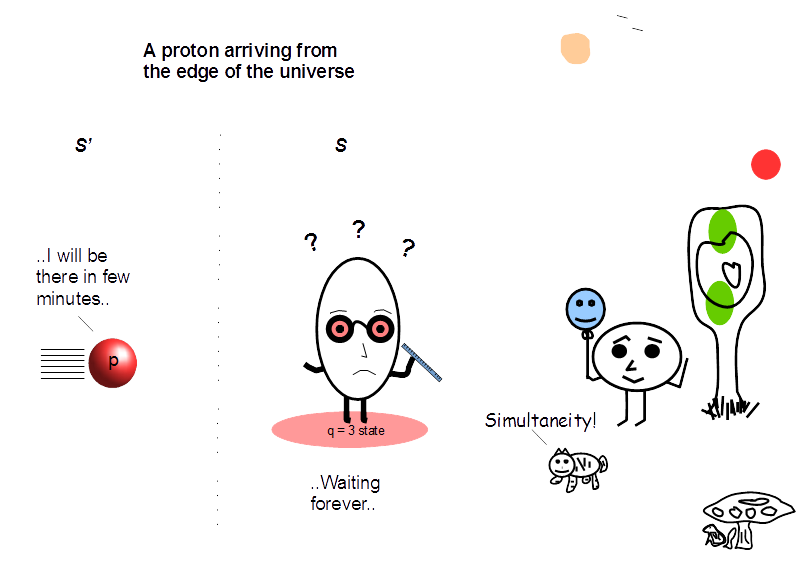Previous Blogs: EPR Paradox-I  Nutshell-2015Information on www.ijspace.org is licensed under a Creative Commons Attribution 4.0 International License. Attribution — You must give appropriate credit, provide a link to the license, and indicate if changes were made. You may do so in any reasonable manner, but not in any way that suggests the licensor endorses you or your use. No additional restrictions — You may not apply legal terms or technological measures that legally restrict others from doing anything the license permits. This is a human-readable summary of (and not a substitute for) the license.